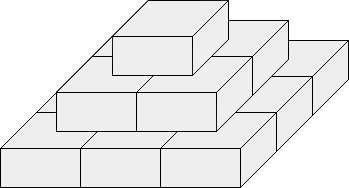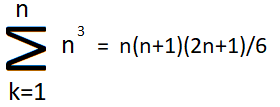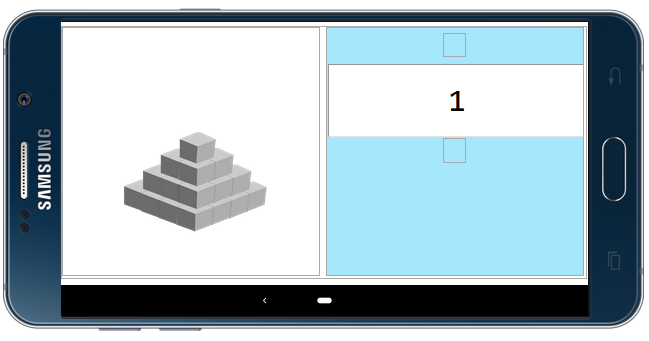# How to calculate stones in a step pyramid block?

How to calculate stones in a step pyramid block.
How can I make a calculator to calculate how many stones or blocks are in a pyramid.In this image have total 14 block.

I want to just enter number of layer in a textbox and two side bottom layer’s blocks number in two different textbox
And calculator give result 14 Blocks.

How can I make this in kodular?
Please anybody help me giving idea or aia.

How much will you pay for an aia?

I want help no paid service.

that’s some simple math, isn’t it?
1 layer = 1 x 1 = 1
2 layer = 1 layer + 2 x 2 = 1 + 4 = 5
3 layer = 2 layer + 3 x 3 = 5 + 9 = 14
4 layer = 3 layer + 4 x 4 = 14 + 16 = 30
etc

Taifun

2 Likes

Yeah, But how can I edit block in kodular ?
I want to make something like that I just entered 3 value of a pyramid.
Where,
1 Value is Number layer of pyramid.
2 Value is Number of total Blocks of One Side Bottom layer.
3 Value is Number of Blocks of Another One Side Bottom layer.

And then give result total number of Blocks have in pyramid.

Is it possible .?

This topic sounded like a student’s homework to me.But I wanted to share it for the benefit of someone else, or a teacher in particular.If you know the formula above:

Design page:Block page:

Images were produced from a single block.

Phone screen shot.

1 Like

Yes exactly

I guess, the teacher is not very happy, that the students can now just copy your solution without learning anything themselves now…

Taifun

Thanks For Your Help But I am totally newbe in this platform I am a Textile Technician. I am trying to make a android app that make my work faster.

In this image have a yarn pyramid stock. I need to calculate all yarn package daily. Which need more time but I want to make calculator type app where I will enter 3 value in 3 textbox that’s I marked on this image and app give me the result how many yarn package have on this pyramid.
I really didn’t understand how can make this.